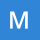Home Communities
IT Knowledge
Inspiration
Languages
EN

# MySQL - difference between two time values

0 points
Created by:Mohammad-Oneal
237

In this article, we would like to show you how to calculate the difference between two time values in MySQL.

Quick solution:

``````-- SELECT TIMEDIFF('to_time','from_time');
SELECT TIMEDIFF('HH:MM:SS','HH:MM:SS');``````

Where:

• `HH` - hour number (from `00` to `24`),
• `MM` - minute number (from `00` to `59`),
• `SS` - second number (from `00` to `59`).

## Practical example

In this example, we will calculate the difference between two time values using `TIMEDIFF()` function.

Query:

``SELECT TIMEDIFF('09:30:00','08:00:00') AS 'time difference';``

Result:MySQL - result of computing the difference between two time values